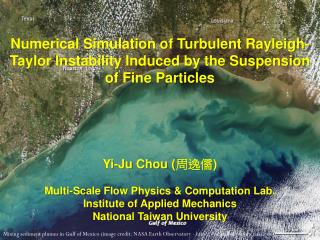DownloadDownload PresentationMixing sediment plumes in Gulf of Mexico (image credit: NASA Earth Observatory - http://earthobservatory.nasa.gov)

# Mixing sediment plumes in Gulf of Mexico (image credit: NASA Earth Observatory - http://earthobservatory.nasa.gov)

Télécharger la présentation## Mixing sediment plumes in Gulf of Mexico (image credit: NASA Earth Observatory - http://earthobservatory.nasa.gov)

- - - - - - - - - - - - - - - - - - - - - - - - - - - E N D - - - - - - - - - - - - - - - - - - - - - - - - - - -
##### Presentation Transcript

1. Numerical Simulation of Turbulent Rayleigh-Taylor Instability Induced by the Suspension of Fine Particles Yi-Ju Chou(周逸儒) Multi-Scale Flow Physics & Computation Lab. Institute of Applied Mechanics National Taiwan University Mixing sediment plumes in Gulf of Mexico (image credit: NASA Earth Observatory - http://earthobservatory.nasa.gov)

2. Sediment River Plume • Ocean acidification • Phytoplankton bloom • Subaqueous ecology Parsons et al, 2001 image credit: NASA Earth Observatory - http://earthobservatory.nasa.gov)

3. Outline • Background and motivation • Mathematical formulation • Numerical examples of RT instability • Summary

4. Physics Background • Important characteristics • Particle diameter ~ O(1-10 m) • Density ratio~ O(1) • Turbulent flow • Concentration (volume fraction) ~ O(0.01) • Important parameters • Particle Reynolds number: < O(100) Stokes drag • Particle relaxation(response) time: sec • Stokes number: • Bagnold number: Collision negligible

5. Physics Background Modeling strategy for dispersed two-phase turbulent flows : Balachandar & Eaton, Annual Rev. Fluid Mech., 2010

6. Review of Existing Method • Single-phase method: Equilibrium state: A balance between drag and gravitational force Scalar limit: Zero-volume for particles Has been employed to study a number of problems related to fine suspensions using DNS, LES, RANS

7. Motion of a Sediment Grain + Basset history term + Saffman lift force Drag Pressure Gravity Added mass : Density ratio : Added-mass coefficient (1/2 for the sphere) : Particle relaxation time

8. Motivation What are we trying to answer? • How can the equilibrium state be a good approximation? • What else are we missing, and how they effect bulk mixing? • Can we improve the current model without too much extra computational effort?

9. Mathematical Formulation • A two-way coupled Euler-Euler solid-liquid system

10. Mathematical Formulation • A two-phase fractional-step pressure projection method Non-Boussinesq pressure Poison solver: Corrector: Pressure projection

11. Mathematical Formulation Differs from traditional models for solid-gas systems in • Three-dimensional turbulence-resolving two-flowsystem (LES, DNS) • Added mass effect (Auton, 1988) • Mixture incompressibility (a two-phase pressure projection method)

12. Mathematical Formulation What are we missing? (Chou et al., 2013b) • Non-equilibrium particle inertia (NEPI) • NEPI effect in the carrier flow (continuous phase) • Mixture incompressibility

13. Particle Induced RT Instability -- A numerical study of two-phase effect in suspensions Simulation setup (Chou et al., 2013b) • = 0.0032, 0.0128, 0.0512 • ; sec • Direct Numerical Simulation (DNS) • BC: Periodic at horizontal; • Solid wall at bottom; • No sediment supply at top. 0.12 m (192) 0.08 m (128) 0.08 m (128)

14. Growth of Initial Perturbations

15. Large-Scale Mixing

16. Growth of mixing zone • Self-similar solution: h

17. Energy Spectrum

18. Energetic Slightly higher energy releaseinduced by non-equilibrium particle inertia

19. Energetic Feedbacks to the carrier flow are increasingly important

20. Energetic Due to mixture incompressibility * * 2-phase modeling without mixture incompressibility

21. Summary • We aim to investigate effects of missing mechanisms induced by two-phase interactions in the common modeling approach for sediment suspension problems. • The two-phase effects include: • -- non-equilibrium particle inertia (NEPI); • -- NEPI in the carrier fluid; • -- mixture incompressibility (MI) • A series of numerical experiments of RT reveals that • -- In low volume fraction, NEPI slightly enhances the energy budget. • -- As concentration increases, NEPI and MI become increasing • important, which suppress energy. • -- MI is significant to suppress energy budget at high concentration, • which accounts for almost ¼ of the reduction of the PE release.

22. Thank you